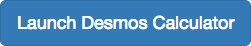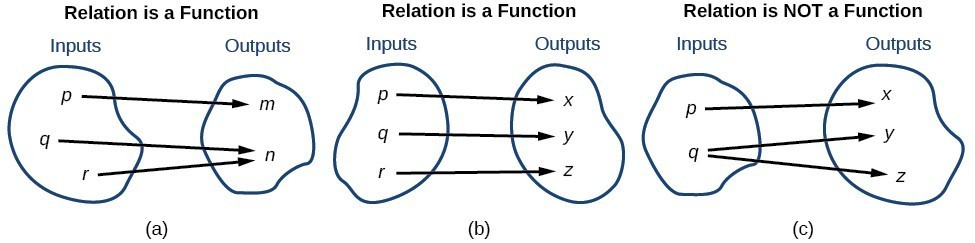## Characteristics of Functions

### Learning Outcomes

• Identify functions given in tabular form.
• Write and evaluate functional relationships using standard notation.
• Determine whether a function is one-to-one.A relation is a set of ordered pairs. The set of the first components of each ordered pair is called the domain of the relation and the set of the second components of each ordered pair is called the range of the relation. Consider the following set of ordered pairs. The first numbers in each pair are the first five natural numbers. The second number in each pair is twice the first.

$\left\{\left(1,2\right),\left(2,4\right),\left(3,6\right),\left(4,8\right),\left(5,10\right)\right\}$

The domain is $\left\{1,2,3,4,5\right\}$. The range is $\left\{2,4,6,8,10\right\}$.

Note the values in the domain are also known as an input values, or values of the independent variable, and are often labeled with the lowercase letter $x$. Values in the range are also known as an output values, or values of the dependent variable, and are often labeled with the lowercase letter $y$.

A function $f$ is a relation that assigns a single value in the range to each value in the domain. In other words, no $x$-values are used more than once. For our example that relates the first five natural numbers to numbers double their values, this relation is a function because each element in the domain, $\left\{1,2,3,4,5\right\}$, is paired with exactly one element in the range, $\left\{2,4,6,8,10\right\}$.

Now let’s consider the set of ordered pairs that relates the terms “even” and “odd” to the first five natural numbers. It would appear as

$\left\{\left(\text{odd},1\right),\left(\text{even},2\right),\left(\text{odd},3\right),\left(\text{even},4\right),\left(\text{odd},5\right)\right\}$

Notice that each element in the domain, $\left\{\text{even,}\text{odd}\right\}$ is not paired with exactly one element in the range, $\left\{1,2,3,4,5\right\}$. For example, the term “odd” corresponds to three values from the domain, $\left\{1,3,5\right\}$ and the term “even” corresponds to two values from the range, $\left\{2,4\right\}$. This violates the definition of a function, so this relation is not a function.

This image compares relations that are functions and not functions.(a) This relationship is a function because each input is associated with a single output. Note that input $q$ and $r$ both give output $n$. (b) This relationship is also a function. In this case, each input is associated with a single output. (c) This relationship is not a function because input $q$ is associated with two different outputs.

### A General Note: FunctionS

A function is a relation in which each possible input value leads to exactly one output value. We say “the output is a function of the input.”

The input values make up the domain, and the output values make up the range.

### How To: Given a relationship between two quantities, determine whether the relationship is a function.

1. Identify the input values.
2. Identify the output values.
3. If each input value leads to only one output value, the relationship is a function. If any input value leads to two or more outputs, the relationship is not a function.

### Example: Determining If Menu Price Lists Are Functions

The coffee shop menu consists of items and their prices.

1. Is price a function of the item?
2. Is the item a function of the price?### Example: Determining If Class Grade Rules Are Functions

In a particular math class, the overall percent grade corresponds to a grade point average. Is grade point average a function of the percent grade? Is the percent grade a function of the grade point average? The table below shows a possible rule for assigning grade points.

 Percent Grade Grade Point Average 0–56 57–61 62–66 67–71 72–77 78–86 87–91 92–100 0.0 1.0 1.5 2.0 2.5 3.0 3.5 4.0

### Try It

The table below lists the five greatest baseball players of all time in order of rank.

Player Rank
Babe Ruth 1
Willie Mays 2
Ty Cobb 3
Walter Johnson 4
Hank Aaron 5
1. Is the rank a function of the player name?
2. Is the player name a function of the rank?

## Using Function Notation

Once we determine that a relationship is a function, we need to display and define the functional relationships so that we can understand and use them, and sometimes also so that we can program them into computers. There are various ways of representing functions. A standard function notation is one representation that makes it easier to work with functions.

To represent “height is a function of age,” we start by identifying the descriptive variables $h$ for height and $a$ for age. The letters $f,g$, and $h$ are often used to represent functions just as we use $x,y$, and $z$ to represent numbers and $A,B$, and $C$ to represent sets.

\begin{align}&h\text{ is }f\text{ of }a &&\text{We name the function }f;\text{ height is a function of age}. \\ &h=f\left(a\right) &&\text{We use parentheses to indicate the function input}\text{. } \\ &f\left(a\right) &&\text{We name the function }f;\text{ the expression is read as }''f\text{ of }a''. \end{align}

Remember, we can use any letter to name the function; we can use the notation $h\left(a\right)$ to show that $h$ depends on $a$. The input value $a$ must be put into the function $h$ to get an output value. The parentheses indicate that age is input into the function; they do not indicate multiplication.

We can also give an algebraic expression as the input to a function. For example $f\left(a+b\right)$ means “first add $a$ and $b$, and the result is the input for the function $f$.” We must perform the operations in this order to obtain the correct result.

### A General Note: Function Notation

The notation $y=f\left(x\right)$ defines a function named $f$. This is read as $y$ is a function of $x.''$ The letter $x$ represents the input value, or independent variable. The letter $y$, or $f\left(x\right)$, represents the output value, or dependent variable.

### Example: Using Function Notation for Days in a Month

Use function notation to represent a function whose input is the name of a month and output is the number of days in that month.

### Example: Interpreting Function Notation

A function $N=f\left(y\right)$ gives the number of police officers, $N$, in a town in year $y$. What does $f\left(2005\right)=300$ represent?

### Q & A

Instead of a notation such as $y=f\left(x\right)$, could we use the same symbol for the output as for the function, such as $y=y\left(x\right)$, meaning “y is a function of x?”

Yes, this is often done, especially in applied subjects that use higher math, such as physics and engineering. However, in exploring math itself we like to maintain a distinction between a function such as $f$, which is a rule or procedure, and the output $y$ we get by applying $f$ to a particular input $x$. This is why we usually use notation such as $y=f\left(x\right),P=W\left(d\right)$, and so on.

## Representing Functions Using Tables

A common method of representing functions is in the form of a table. The table rows or columns display the corresponding input and output values. In some cases these values represent all we know about the relationship; other times the table provides a few select examples from a more complete relationship.

The table below lists the input number of each month (January = 1, February = 2, and so on) and the output value of the number of days in that month. This information represents all we know about the months and days for a given year (that is not a leap year). Note that, in this table, we define a days-in-a-month function $f$, where $D=f\left(m\right)$ identifies months by an integer rather than by name.

 Month number, $m$ (input) 1 2 3 4 5 6 7 8 9 10 11 12 Days in month, $D$ (output) 31 28 31 30 31 30 31 31 30 31 30 31

The table below defines a function $Q=g\left(n\right)$. Remember, this notation tells us that $g$ is the name of the function that takes the input $n$ and gives the output $Q$.

 $n$ 1 2 3 4 5 $Q$ 8 6 7 6 8

The table below displays the age of children in years and their corresponding heights. This table displays just some of the data available for the heights and ages of children. We can see right away that this table does not represent a function because the same input value, 5 years, has two different output values, 40 in. and 42 in.

 Age in years, $\text{ }a\text{ }$ (input) 5 5 6 7 8 9 10 Height in inches, $\text{ }h\text{ }$ (output) 40 42 44 47 50 52 54

### How To: Given a table of input and output values, determine whether the table represents a function.

1. Identify the input and output values.
2. Check to see if each input value is paired with only one output value. If so, the table represents a function.

### Example: Identifying Tables that Represent Functions

Which table, A, B, or C, represents a function (if any)?

Table A
Input Output
2 1
5 3
8 6
Table B
Input Output
–3 5
0 1
4 5
Table C
Input Output
1 0
5 2
5 4

When we know an input value and want to determine the corresponding output value for a function, we evaluate the function. Evaluating will always produce one result because each input value of a function corresponds to exactly one output value.

When we know an output value and want to determine the input values that would produce that output value, we set the output equal to the function’s formula and solve for the input. Solving can produce more than one solution because different input values can produce the same output value.

## Determine whether a function is one-to-oneSome functions have a given output value that corresponds to two or more input values. For example, in the following stock chart the stock price was $1000 on five different dates, meaning that there were five different input values that all resulted in the same output value of$1000.

However, some functions have only one input value for each output value, as well as having only one output for each input. We call these functions one-to-one functions. As an example, consider a school that uses only letter grades and decimal equivalents, as listed in.

A 4.0
B 3.0
C 2.0
D 1.0

This grading system represents a one-to-one function, because each letter input yields one particular grade point average output and each grade point average corresponds to one input letter.

To visualize this concept, let’s look again at the two simple functions sketched in (a) and (b) below.The function in part (a) shows a relationship that is not a one-to-one function because inputs $q$ and $r$ both give output $n$. The function in part (b) shows a relationship that is a one-to-one function because each input is associated with a single output.

### A General Note: One-to-One Function

A one-to-one function is a function in which each output value corresponds to exactly one input value.

### Example: Determining Whether a Relationship Is a One-to-One Function

Is the area of a circle a function of its radius? If yes, is the function one-to-one?

### Try It

1. Is a balance a function of the bank account number?
2. Is a bank account number a function of the balance?
3. Is a balance a one-to-one function of the bank account number?

## Contribute!

Did you have an idea for improving this content? We’d love your input.